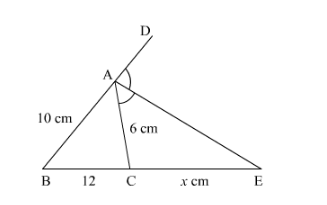# In Fig. 4.57, AE is the bisector of the exterior ∠CAD meeting BC produced in

Question:

In Fig. 4.57, AE is the bisector of the exterior ∠CAD meeting BC produced in E. If AB = 10 cm, AC = 6 cm and BC = 12 cm, find CE.Solution:

It is given that $A E$ is the bisector of the exterior $\angle C A D$

Meeting $B C$ produced $E$ and $A B=10 \mathrm{~cm}, A C=6 \mathrm{~cm}$ and $B C=12 \mathrm{~cm}$

Since $A E$ is the bisector of the exterior $\angle C A D$

So $\frac{B E}{C E}=\frac{A B}{A C}$

$\frac{12+x}{x}=\frac{10}{6}$

$72+6 x=10 x$

$4 x=72$

$x=18$

Hence $C E=18 \mathrm{~cm}$